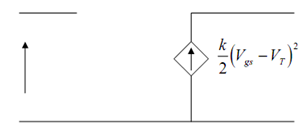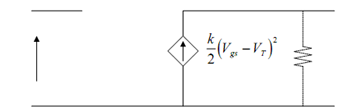## Small Signal Model Assignment Help

Assignment Help: >> Metal-Oxide-Semiconductor Field Effect Transistor - Small Signal Model

Small Signal Model:

This shall be easy to make a small signal model for MOSFET for AC analysis by drawing analogy with the small signal model of a BJT. The drain behaves like a current source, which is controlled by Vgs. What must we do about the gate terminal? Actually the gate is not connected to anything inside the transistor, so this looks just like an open circuit. (Actually, there is a capacitance Cgate = Cox Agate, where Agate = W L, the area of the gate, however in most low frequency linear applications, this capacitance is not significant.) Therefore, our small signal model for the MOSFET, if this is operating in it saturation mode, is as illustrated in FigureFigure: Small Signal MOSFET Model

This seems to be a good amplifier. It has infinite input impedance (and therefore shall not load down the previous stage of the amplifier) and it has a non-linear voltage controlled current source for its output. As Vds is enhanced, the channel length gets shorter. The enhanced Vds makes the pinch off region expands. A shorter channel means somewhat less channel resistance, and so Id in fact increases with increasing Vds rather than staying constant. We saw through the bipolar transistor, that while this occurs, we should add a resistor in parallel with our current source. Therefore, let's complete the model with an additional r0 but, we shall put it in with a dashed line, because except for extremely short channel devices, it has extremely little effect on device performance as in Figure.